# NCERT Class 9 Science Notes & Solutions – Chapter 8 – Motion

## Exercises Questions & Answers - Motion Class 9 Science NCERT

#### In-Text Questions & Answers | NCERT Chapter 8 | Motion Class 9 Science

##### In-Text Questions & Answers | NCERT Chapter 8 | Motion Class 9 Science | Page Nos. 100

1. An object has moved through a distance. Can it have zero displacement? If yes, support your answer with an example?
Off course, it is possible. If the the starting or ending point of a journey is same, then total displacement is zero. For example, someone goes to Mumbai from Kolkata & returns back to Kolkata. Though he covers some distance but displacement is zero. Similarly, an object starts journey along a circular path & completes one revolution. After one revolution the object returns to the  same point where it started the journey. So, the net displacement is zero.

##### 2. A farmer moves along the boundary of a square field of side 10 m in 40 s. What will be the magnitude of displacement of the farmer at the end of 2 minutes 20 seconds from his initial position?

Let us consider the square as ABCD. So, AB=BC=CD=DA= 10 m. Total perimeter = 4 x 10 m = 40 m. Speed = (40/40) m = 1 m/s. Distance covered by the farmer in 2 m 20 s i.e. (60×2 + 20 = 140 s) = [ 1 x 140 ] m = 140 m = [3×40  + 20 ] m = 3 rotation  +   20 m. So the farmer completes 3 rotation & covers another 20 m. Now, 20 m from A is the C point. Therefore, displacement is AC.
AC = √(AB2 + BC2) = √(102 + 102) = √200 = 10√2 m.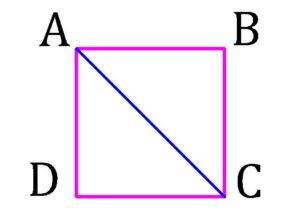3. Which of the following is true for displacement?
a) It cannot be zero.
b) Its magnitude is greater than the distance travelled by the object.

a) Displacement may be zero if starting & ending positions are same. So the statement is not true.
b) Displacement is the measurement between initial & final position. It does not get affected by the distance covered by the object. It only measures the distance between the initial & final position of the object. So the statement is not true.

##### In-Text Questions & Answers | NCERT Chapter 8 | Motion Class 9 Science | Page Nos. 102

1. Distinguish between speed & velocity.

Speed

Velocity

Speed is the distance covered by a moving object in a second.
Velocity is the rate of the change of the displacement with the time.
Scalar quantity.
Vector quantity.
Basically it is velocity without direction.
Basically it is speed with direction.
It may or may not be equal to velocity.
A body may possesses different velocities but the same speed.

2. Under what condition is the magnitude of average velocity of an object equal to its average velocity?

If the velocity is uniform along a straight line, then the magnitude of average velocity of an object equal to its average velocity. Because only in this condition, total distance covered & displacement is equal.
Average velocity = Displacement/Time.
Average speed = Total distance covered/Time.
So, when displacement & total distance covered are equal, then average velocity & average speed are equal.

##### 3. What does the odometer of an automobile measure?

It measures the distance travelled by an automobile. Its technology is based on the perimeter of the wheel as the wheel rotates during the motion of the automobile.

4. What does the path of an object look like when it is in uniform motion?
The path will look like a straight line. Because uniform motion is a motion in which the body covers equal distance in equal intervals of time along a straight line.

5. During an experiment, a signal from a spaceship reached the ground station in five minutes. What was the distance of the spaceship from the ground station? The signal travels at the speed of light i.e. 3 x 108 m s-1.
At first => Distance = Speed x Time.
In this case, speed = 3 x 108 ms-1 & time = 5 Minutes = 5 x 60 Seconds = 300 Seconds.
So, the distance = 3 x 108 x 300 m = 9 x 1010 m.

##### In-Text Questions & Answers | NCERT Chapter 8 | Motion Class 9 Science | Page Nos. 103

1. When will you say a body is in ⇒ a) uniform acceleration? b) non-uniform acceleration?
a) Uniform acceleration ⇒ When the motion of the body is along a straight line & its velocity is changing by equal magnitude in equal interval of time.
b) Non-uniform acceleration ⇒ When the motion of the body is along a straight line & its velocity is changing by unequal magnitude in equal interval of time.

2. A bus decreases its speed from 80 km h-1 to 60 km h-1 in 5 s. Find the acceleration of the bus.
We know, acceleration = a = (v-u)/t. [From Equation 4]
Here, u = 80 km h-1 = (80×1000)/3600 ms-1 = 200/9 ms-1.
v = 60 km h-1 = (60×1000)/3600 ms-1 = 150/9 ms-1. t = 5 s.
So, a = [(150/9) – (200/9)] / 5  ms-2 = – 1.1 ms-2. The (-) sign indicates that it is negative acceleration or deceleration.

3. A train starting from a railway station & moving with uniform acceleration attains a speed 40 km/h in 10 minutes. Find its acceleration.
In this case, a = (v-u)/t. Here, u=0, v = 40 km h-1 = (40×1000)/3600 ms-1 = 100/9 ms-1. t=10 minute =600 s. So, a = [(100/9) – 0]/600  ms-2 = 0.0185 ms-2.

##### In-Text Questions & Answers | NCERT Chapter 8 | Motion Class 9 Science | Page Nos. 107

1. What is the nature of the distance-time graphs for uniform & non-uniform motion of an object?
The nature of the distance-time graphs for uniform motion of an object is a straight line. The nature of the distance-time graphs for non-uniform motion of an object is not a straight line.

2. What can you say about the motion of an object whose distance-time graph is a straight line parallel to the time axis?
The object is stationary as the graph is straight line & parallel to the time axis due to not changing its position.

3. What can you say about the motion of an object if its speed-time graph is a straight line parallel to the time axis?
The object is in uniform motion as the graph is straight line & parallel to the time axis due to not changing in speed with time.

4. What is the quantity which is measured by the area occupied below velocity-time graph?
It measures the distance.

##### In-Text Questions & Answers | NCERT Chapter 8 | Motion Class 9 Science | Page Nos. 109-110

1. A bus starting from rest moves with a uniform acceleration of 0.1 m s-2 for 2 minutes. Find ⇒ a) the speed acquired. b) the distance travelled.
a) In this case, v = u + at , u = 0, a = 0.1 ms-2, t = 2 minutes  = 2×60 s = 120 s, v = ??.
So, v =[ 0  +  0.1×120] m/s = 12 m/s.

b) In this case, s = ut + (1/2)at2 = [0x120 + (1/2)x0.1×1202 ] m = 720 m.

2. A train is travelling at a speed of 90 km h-1. Brakes are applied so as to produce a uniform acceleration of -0.5 m s-2. Find how far the train will go before it is brought to rest.
We know, v2  = u2 + 2as. In this case, u = 90 km/h = (90×1000)/3600 m/s = 25 m/s, a = -0.5 ms-2, s  = ?
So, v2  = u2 + 2as,
So, 02 = [252 + 2x(-0.5)xs] ,
⇒ s = 625 m.

3. A trolley, while going down an inclined plane, has an acceleration of 2 cm s-2. What will be its velocity 3 s after the start?
We know, v  = u + at. In this case, u = 0, a = 2 cms-2 = 0.02 ms-2 , t = 3 seconds, v = ?
So, v = [0 + 3×0.02] m = 0.06 m/s.

##### 4. A racing car has a uniform acceleration of 4 m s-2. What distance will it cover in 10 s after start?

We know, s = ut + (1/2)at2. In this case, u = 0, a = 4 ms-2, t = 10 seconds, s = ?
So, s = [0x10  +  (1/)x4x102 ] m = 200 m.

5. A stone is thrown in a vertically upward direction with a velocity of 5 m s-1. If the acceleration of the stone during its motion is 10 m s-2 in the downward direction, what will be the height attained by the stone & how much time will it take to reach there?
We know, v2  = u2 + 2as. In this case, u = 5 m/s, a = -10 ms-2 , v = 0, seconds, s = ?
So, 02 = [52 + 2x(-10)xs] ,
⇒ s = [25/20] m = 1.25 m.

Again,  v = u + at ,
⇒ t = (v-u)/a  =  [(0-5)/ (-10)] s = 0.5 s.
Therefore, the stone attains a height of 1.25 m in 0.5 s.

##### Exercises Questions & Answers | NCERT Chapter 8 | Motion Class 9 Science | Page Nos. 112-113
###### 1. An athlete completes one round of a circular track of diameter 200 metre in 40 seconds. What will be the distance covered and the displacement at the end of 2 minutes 20 seconds?

Athlete walks along the perimeter or circumference of the circle. Now, the perimeter of the circle = 2πr (where π = 22/7, r = radius of circle)
2πr = π x 2r = π x R  (R = 2r = diameter of circle). Therefore, perimeter = [22/7] x 200 m = [ 4400/7 ] m.
So, the speed = [ 4400/7] / 40 m/s [ as athlete completes one round i.e. perimeter in 40 second].
⇒ 4400 / (7 x 40) m/s = 110/7  m/s.
Also 2 minutes 20 seconds = [ (2 x 60) + 20 ] seconds = 140 seconds. Distance Covered at the end of 2 minutes 20 seconds i.e. 140 seconds
⇒ Speed x Time =  ( 110/7 ) x 140  metre =   2200 metre.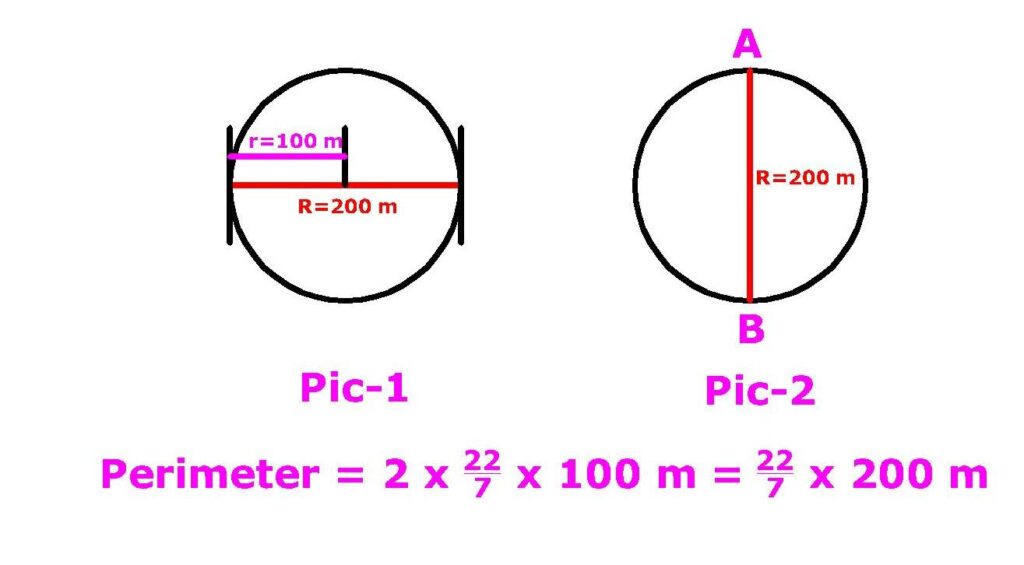Displacement at the end of 2 minutes 20 seconds i.e. 140 seconds: Displacement is the distance between start point and end point. Athlete completes one round in 40 seconds. So after every 40 seconds athlete reaches same point where he started. So after every 40 seconds displacement is zero. Now 140 seconds = [ 40 + 40 + 40 + 20 ] s = [(3 x 40 ) + 20 s].

We see that in total 140 seconds athlete makes 3 complete round which takes 120 seconds. After that we will find his location at 140 s i.e. 20 s after 120 s. Then we will measure distance between his location and starting point which is the actual displacement. Athlete completes one round in 40 seconds, so obviously in 20 secs athlete will complete ½ round which is shown in Picture-2. Athlete reaches from A to B. So displacement will be AB i.e. diameter of the circle  which is 200 metre.

###### 2. Joseph jogs from one end A to other end B of a straight 300 m road in 2 minutes 30 seconds and then turns around and jogs 100 m back to point C in another 1 minute. What are Josephs average speeds and velocities in jogging (a) from A to B and (b) from A to C?

a) 2 minutes 30 seconds = 2 x 60 + 30 seconds = 150 seconds.

average speed = distance covered/time = [ AB/ 150 ] m/s= [300/150] m/s = 2 m/s.
average velocity = displacement/time = [ AB/ 150 ] m/s= [300/150] m/s = 2 m/s

b) 2 minutes 30 seconds + 1 minute = [(2 x 60 + 30) + 60] seconds = 210 seconds.
average speed = distance covered/time = [ (AB+CB)/ 210 ] m/s= [400/210] m/s =1.90 m/s.
average velocity = displacement/time = [ AC/ 210 ] m/s= [200/150] m/s = 0.95 m/s.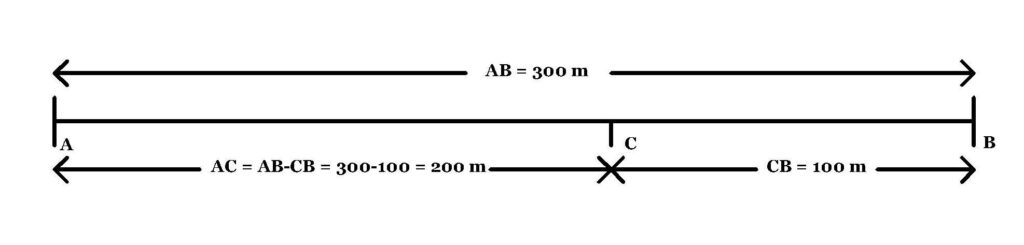In this case displacement is AC i.e. distance between starting point and ending point.

###### 3. Abdul, while driving to school, computes the average speed for his trip to be 20 km/h. On his return trip along the same route, there is less traffic and the average speed was 30 km/h. What is the average speed for Abdul’s trip?

Here assume distance is D km.
Time taken to go to school = T1 = (Distance/Speed) = (D/20) hr.
Time taken to return from school = T2 = (Distance/Speed) = (D/30) hr.
Abdul goes to school and returns from school. So the distance covered by him is (D+D)km = 2D km.

Therefore, average speed = [ total distance covered / total time ] = [ 2D /(T1 + T2) ]
=[ 2D / ( D/20  + D/30 ) ] kmh-1 = 24 kmh-1 .

Q4. A motorboat starting from rest on a lake accelerates in a straight line at constant rate of 3.0 ms-2 for 8.0 s. How far does the boat travel during this time?
We know distance = S = ut + (½)at2 . In this case, u = initial velocity = 0, t = 8 s, a = 3.0 ms-2.
So, distance = S = [ 0 x 8  +  (½) x 3 x 82 ] m = 96 m.

###### Q5. A driver of a car travelling at 52 km/hr applies the brakes & accelerates uniformly in the opposite direction. The car stops in 5 s. Another driver going at 3 km/hr in another car applies his brakes slowly & stops in 10 s. On the same graph paper plot the speed vs time graphs for the two cars. Which of the two cars travelled farther after the brakes were applied?

Observe the following graph.
1st car speed = 52 km/hr = (52000/3600) m/s = 14.444 m/s.
So, the distance covered by the 1st car = Area of triangle ABC = (1/2) x AB x AC = (1/2) x 14.444 x 5 m = 36.11 m.
2nd car speed = 3 km/hr = (3000/3600) m/s = 0.833 m/s.
So, the distance covered by the 2nd car = Area of triangle ADC = (1/2) x AD x AE = (1/2) x 0.833 x 10 m = 4.16 m.
Therefore, 1st car travelled farther than the 2nd car after braking.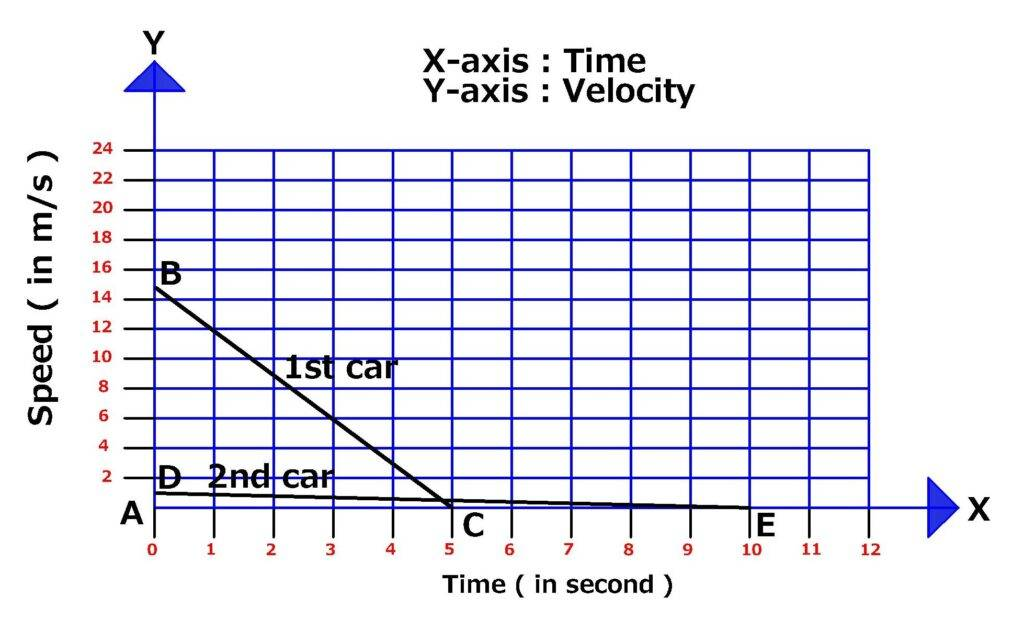###### Q6. Study the following distance-time graph of three objects A, B &amp; C.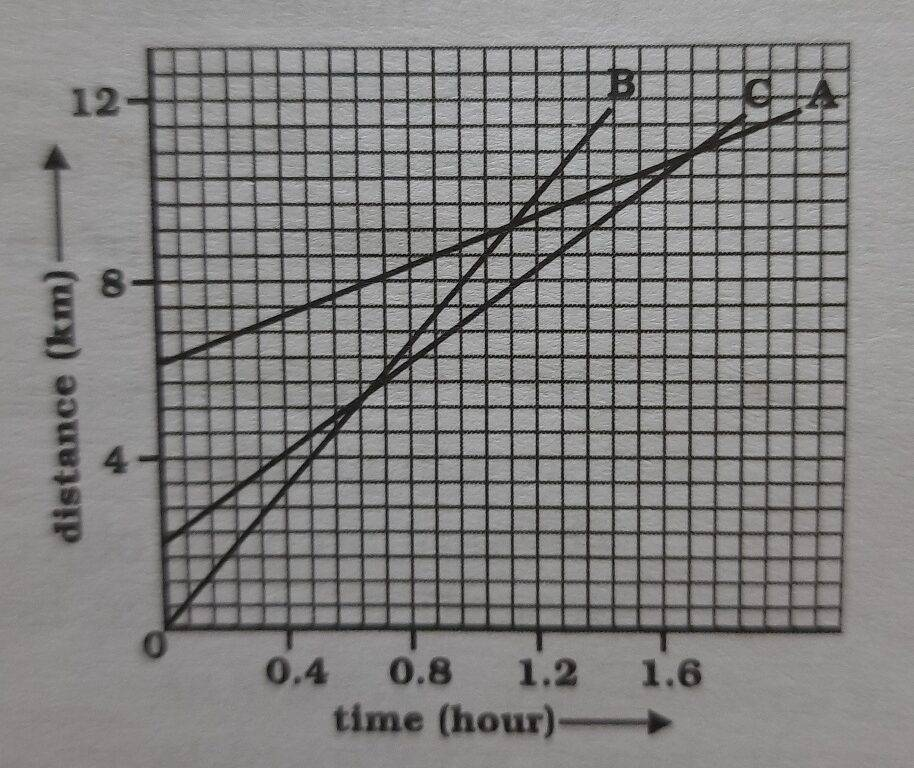a) Which of the three is travelling the fastest?
As B takes less time to cover more distance, so B is travelling fastest.
b) Are all three ever at the same point on the road?
They are never at the same point on the road.
c) How far has C travelled when B passes A?
(8 km – 6 km) = 2 km.
d) How far has B travelled by the time it passes C?
(7 km – 0 km) = 7 km.

###### Q7. A ball is gently dropped from a height of 20 m. If it’s velocity increases uniformly at the rate of 10 m/s2, with what velocity will it strike the ground? After what time will it strike the ground?

Part 1 : We know,  2as = v2 –u2.
In this case, acceleration = a = 10 m s-2, distance = s = 20m, u = initial velocity = 0, v = ?
2as = v2 –u2
⇒ 2x10x20 = v2 –02
⇒ v2 = 400
So, v = 20 ms-1

Part 2 :  We know  v = u + at, ⇒ at = v –u , ⇒ t = (v-u)/a .
In this case, v = 20 ms-1, u = 0, a = 10 m s-2.
So, t = (v-u)/a = ( 20 – 0)/10
⇒ t = 2 s.

###### Q8. The speed-time graph is shown in the following. Study it & answer the followings ⇒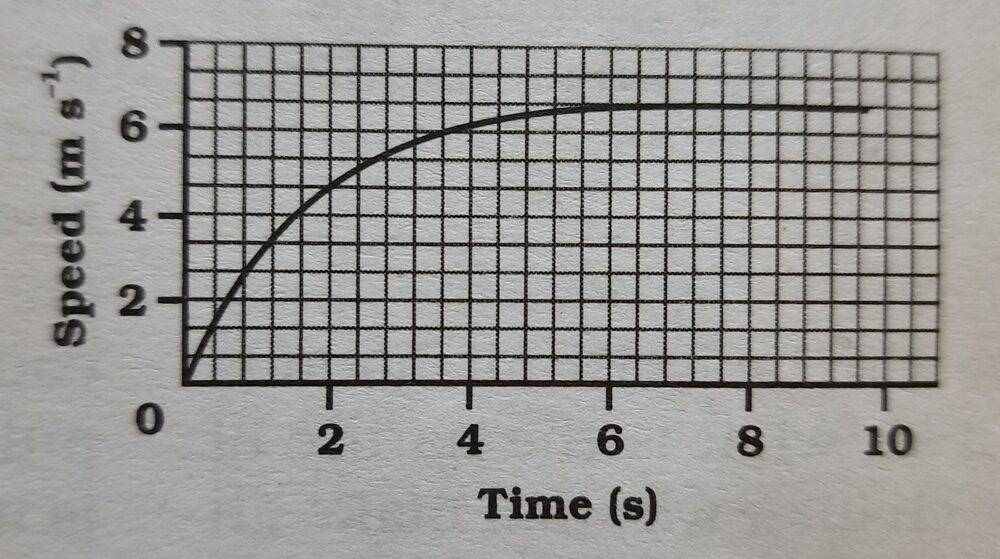a) Find how far does the car travel in the first 4 seconds. Shade the area on the graph that represents the distance travelled by the car during the period?
Time = 4 seconds, Speed = 6 m/s. So distance covered = (1/2) x 4 x 6 m = 12 m.
b) Which part of the graph represents uniform motion of the car?
Motion after 6 seconds shows uniform motion.

###### Q9. State which of the following situations are possible & give an example for each of these ⇒a) an object with a constant acceleration but with zero velocity.

In case of free falling, an object has acceleration when it just starts to fall. This acceleration is due to gravity & equal acceleration due to gravity.

b) an object moving with an acceleration but with uniform speed.

In case of uniform circular motion, an object has to turn in every moment during his journey. So the only change occurs during his motion is the change in direction of motion. Therefore, the motion along a circular path is an accelerated motion.

c) an object moving in a certain direction with an acceleration in the perpendicular direction.

An object moving in a certain direction is acted upon by acceleration due to gravity in the perpendicular direction i.e. vertically downwards.

###### Q10. An artificial satellite is moving in a circular orbit of radius 42250 km. Calculate it’s speed if it takes 24 hours to revolve around the earth?

Circumference or perimeter of the orbit of radius 42250 km around the earth-2xπxr = [2 x (22/7) x 42250] km = 2,65,571.42 km.
The satellite covers 2,65,571.42 km in 24 hrs.
So speed = ( distance/ time) = (2,65,571.42 / 24 ) = 11,065.47 kmh-1 .
Convert it into km/second = (11,065.47/3600) kms-1  = 3.07 kms-1  .
[ 1 hour = 60 minutes = 60×60 seconds = 3600 seconds ]

Motion Class 9 Science – To Be Continued – Motion Class 9 Science